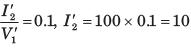Courses

# Test: Two Port Network - 1

## 10 Questions MCQ Test Network Theory (Electric Circuits) | Test: Two Port Network - 1

Description
This mock test of Test: Two Port Network - 1 for Electrical Engineering (EE) helps you for every Electrical Engineering (EE) entrance exam. This contains 10 Multiple Choice Questions for Electrical Engineering (EE) Test: Two Port Network - 1 (mcq) to study with solutions a complete question bank. The solved questions answers in this Test: Two Port Network - 1 quiz give you a good mix of easy questions and tough questions. Electrical Engineering (EE) students definitely take this Test: Two Port Network - 1 exercise for a better result in the exam. You can find other Test: Two Port Network - 1 extra questions, long questions & short questions for Electrical Engineering (EE) on EduRev as well by searching above.
QUESTION: 1

### [T] = ?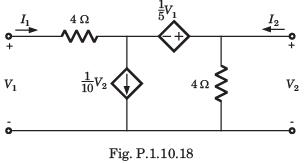Solution:

Let I3 be the clockwise loop current in center loop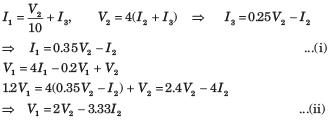QUESTION: 2

### [h] = ?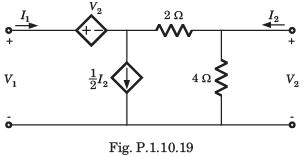Solution: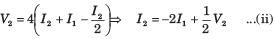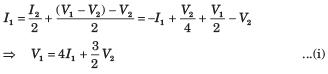QUESTION: 3

### [y] = ?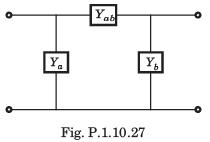Solution: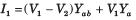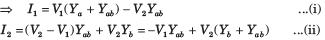QUESTION: 4

The y-parameters of a 2-port network are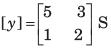A resistor of 1 ohm is connected across as shown in fig. P.1.10.2 8. The new y –parameter would be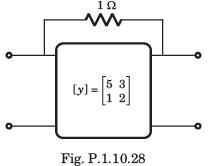Solution:

y-parameter of 1Ω resistor network are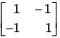New y-parameter =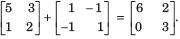QUESTION: 5

For the 2-port of fig. P.1.10.29,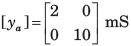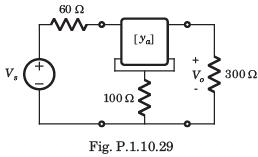The value of Vo/Vs is

Solution: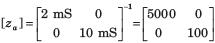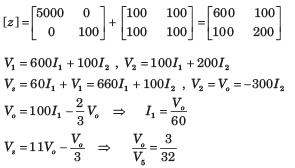QUESTION: 6

The T-parameters of a 2-port network are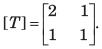If such two 2-port network are cascaded, the z –parameter for the cascaded network is

Solution: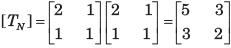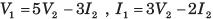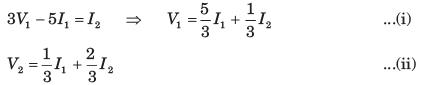QUESTION: 7

[y] = ?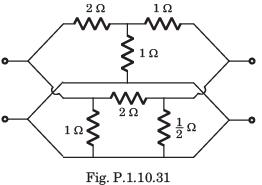Solution: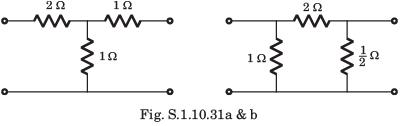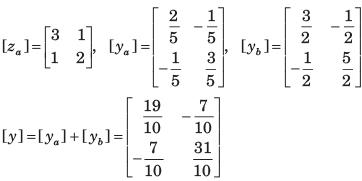QUESTION: 8

[y] = ?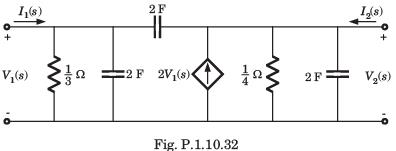Solution: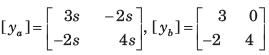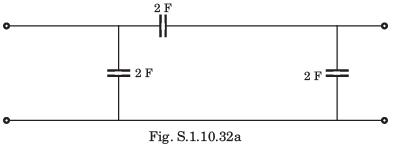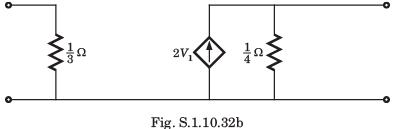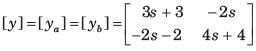QUESTION: 9

h21 = ?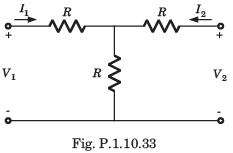Solution: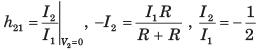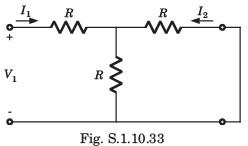QUESTION: 10

In the circuit shown in fig. P.1.10.34, when the voltage V1 is 10 V, the current I is 1 A. If the applied voltage at port-2 is 100 V, the short circuit current flowing through at port 1 will be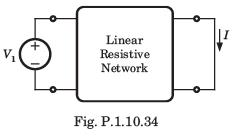Solution: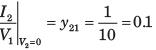Interchanging the port